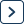JEE  >  Formula Sheets for JEE

# Formula Sheets for JEE - Mathematics For JEE | JEE | Notes, Videos & Tests

Formula Sheets for JEE is topic-wise collection of Important notes, Topic Wise tests, Video lectures, NCERT Textbook, NCERT Solution, and Previous Year papers is designed in a way where you get a complete chapter-wise package for your preparation of Mathematics For JEE in one place? Here, the chapter-wise guide is framed by the best teachers having tremendous knowledge in the respective streams, thereby making the Formula Sheets for JEE - Mathematics For JEE the ultimate study source for the chapter.
Formula Sheets for JEE - Mathematics For JEE | JEE | Notes, Videos & Tests is part of JEE 2023 for Mathematics For JEE preparation. The notes and questions for Formula Sheets for JEE - Mathematics For JEE | JEE | Notes, Videos & Tests have been prepared according to the JEE exam syllabus. Information about Formula Sheets for JEE - Mathematics For JEE | JEE | Notes, Videos & Tests covers all important topics for JEE 2023 Exam. Find important definitions, questions, notes, meanings, examples, exercises and tests below for Formula Sheets for JEE - Mathematics For JEE | JEE | Notes, Videos & Tests. Introduction of Formula Sheets for JEE - Mathematics For JEE | JEE | Notes, Videos & Tests in English is available as part of our Mathematics For JEE for JEE & Formula Sheets for JEE - Mathematics For JEE | JEE | Notes, Videos & Tests in Hindi for Mathematics For JEE course. Download more important topics related with Formula Sheets for JEE - Mathematics For JEE | JEE | Notes, Videos & Tests, notes, lectures and mock test series for JEE Exam by signing up for free.
In this chapter you can find the Formula Sheets for JEE - Mathematics For JEE | JEE | Notes, Videos & Tests defined & explained in the simplest way possible. Besides explaining types of Formula Sheets for JEE - Mathematics For JEE | JEE | Notes, Videos & Tests theory, EduRev gives you an ample number of questions to practice Formula Sheets for JEE - Mathematics For JEE | JEE | Notes, Videos & Tests tests, examples and also practice JEE tests.
 1 Crore+ students have signed up on EduRev. Have you?

## Mathematics For JEE

359 docs | 130 videos | 306 tests

## Notes for Formula Sheets for JEE - Mathematics For JEE | JEE

Formula Sheets for JEE Notes for JEE is part of Mathematics For JEE Notes for Quick Revision. These Formula Sheets for JEE sections for Mathematics For JEE Notes are comprehensive and detailed yet concise enough to glance through for exam preparations. The Formula Sheets for JEE Topic is one of the critical chapters for JEE aspirants to understand thoroughly to perform well in the Mathematics For JEE Section of the JEE Examination. Many aspirants find this section a little complicated and thus they can take help from EduRev notes for JEE, prepared by experts according to the latest JEE syllabus.

## Other Chapters in Mathematics For JEE

The Complete Chapterwise preparation package of Mathematics For JEE is created by the best JEE teachers for JEE preparation. 293414 students are using this for JEE preparation.
Formula Sheets for JEE | Mathematics For JEE
 01 Algebra11 Subtopics02 Coordinate Geometry4 Subtopics03 Trigonometry2 Subtopics04 Calculus7 Subtopics05 Vector Algebra9 Videos  | 10 Docs | 11 Tests06 Statistics & Probability3 Subtopics07 Three Dimensional Geometry7 Videos  | 10 Docs | 15 TestsPart of the course
 Mathematics For JEE 359 docs | 130 videos | 306 tests(Scan QR code)